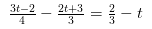# Solve the following linear equations: 3t - 2/4 - 2t + 3/3 = 2/3 -t

Solve the following linear equations: